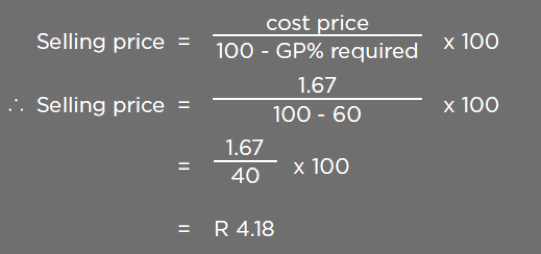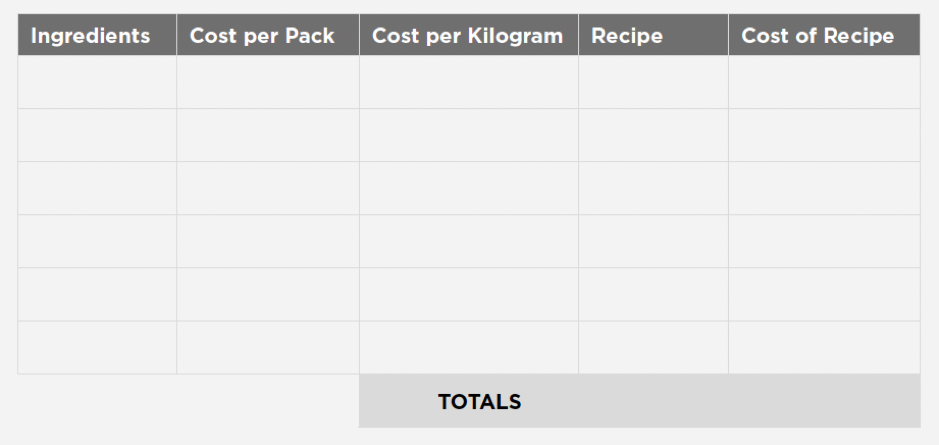### BakingGlossary

#### Recipe Cost Calculator

Basic steps in calculating the selling price:

For example cost price R 1.67
Required GP% of 60%

EquationPlease note: This example of costing excludes utilities and VAT.

Recipe costing exercise

Determine the cost price of the loaf of bread from the following recipe:

Salt = 1.9%
Fat = 2%
Improver = 1%
Yeast = 1.8%
Water = 62%

Raw material costs (excluding VAT)

White Bread Flour – R 7.67 p/kg x 65 kg = R 498.65
Salt – R 1.4992 p/kg x 50 kg = R 74.96
Improver – Not used in this recipe
Yeast – R 10.71 p/litre x 20 L = R 214.20
Water – R 11.40 p/kg x 25 kg = R 285.00

Complete the following table using a flour weight of 50 kg for the recipe:Using a scaling weight of 0.780 kg per loaf of bread, complete the following table:

Yield
Cost per loaf
Cost per loaf including VAT